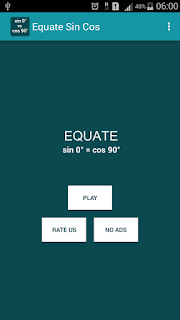## Posts

Showing posts from November, 2016

### Calculate Unknown Variables in Equations with Equation CalculatorEquation Calculator is a tool to calculate math problem by typing the equations. Its will be give you the best numerical approximation of unknown values that contained in the equations. Smaller error value is indicate the approximation is more precision.

For free version, a demo mode, you need to restart this app every 10 times calculating. This feature will let you to try this app, and to know how to use it. If you like this app and willing to no limited usage, you can appreciate and support our work by buy this app.

get Equation Calculator at link below:

### Let Solve Math Linear Equation TestMath Linear Test is simple math game that provided to you several linear math equation. The game play is simple, you just choose what the value must be x,y or z  that suitable with the equation. And the score that you can get is represent to you how far your knowledge about math linear equation with one, two or three unknown variable.

You can get this app free at:

Let try our math game. And have fun!

### Learn and try counting with Counting Bugs GamesCounting Bugs is simple math counter game that purposes to kids. The gameplay is just to count how many the appearing bugs at the top box, then choose how many number of special bugs that asked in the question. If your answer is correct you will get score 10, and less than 10 if your answer in incorrect. All Bug pictures that we use in here is from Greenfoot app and http://pixabay.com

You can get this app at https://play.google.com/store/apps/details?id=com.priantos.countingbugs

### Try Finding Root of Square Equation with Square Root Quiz GamesSquare Root Quiz is simple game that provided to you several square equation. The gameplay is just choose the right answer of x value that correct to the equation. If you choose the correct value for x, you will get score 10, and further more.

You can find this app at link below: